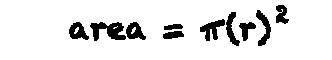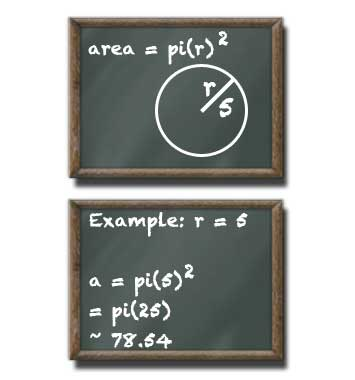# Circle Area Calculator

## Circle Area

#### For Circles with Known Radius

Result:
78.54decimals

A circle with a radius of 5 units has an area of 78.54 square units.

This calculator and more easy to use calculators waiting at www.KylesCalculators.com### Calculating the Area of a Circle:

The area of a circle with a known radius can calculated using the following formula:

A = PI(r)^2

The symbol ^2 means to square the item inside the parentheses, in this case the radius. After the radius has been squared multiply the result by PI. The constant PI can be approximated as 3.14159, but your calculator probably has a button for this.

Below is an example of how to find the area of a circle with the radius of 4. I've used the PI button on my calculator, but you can substitute 3.14159.

• A = pi(4)^2
• = pi(16)
• = 50.2654825
• ~ 50.3

### Radius and Calculating from Diameter

Radius is the measured distance from the centre of a circle to the edge. If you don't know the radius but you do know the total width of the circle finding the radius is a snap. The circle's width is known as diameter. Radius can easily be calculated from diameter by dividing it in half because diameter is passing through the centre of the circle from edge to edge it is twice as large as radius (it is made up of two radii).

Here is an example of calculating radius given a circle with a diameter of 8.

• r = d / 2
• = 8 / 2
• = 4